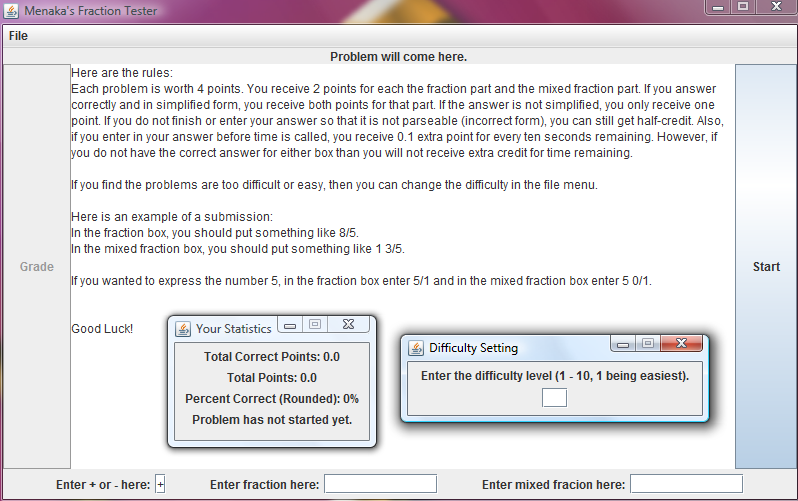# Project: Fraction Tutorial Application

The real motivation for this project, as much as I hate to admit it, was actually my younger sister Menaka. Sometime over the summer my father asked me to help her brush up on her fraction arithmetic skills. So, instead of sitting down with an elementary math book and working some problems with her, I thought I'd write a program which would quiz her.

In essence the program generates random fraction problems of the form

<FRAC1> <OP> <FRAC2>

where the operands are either mixed numbers, proper fractions, or improper fractions, and the operator is either addition, subtraction, multiplication, or division. The program then asks the user for answer to the problem in both mixed fraction / regular fraction form, and grades the response based on accuracy and speed.

In addition, I added a "Difficulty" feature to the program. In the easier levels, the denominators of the fractions don't go above 3 or 4 and the problem is often a trivial fraction multiply, but as you progress the numbers become messier.

Overall, I became very familiar with the Swing GUI Libraries and AWT's FlowLayout and BorderLayout, and of course brushed up quite a bit on my Java. That being said, I think my sister might've used the program maybe once or twice before abandoning it for a more traditional textbook. Oh well.Click the picture above to run the Fraction Tutorial Application.